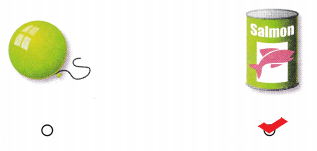# Texas Go Math Kindergarten Lesson 18.1 Answer Key Identify Cylinders

Refer to our Texas Go Math Kindergarten Answer Key Pdf to score good marks in the exams. Test yourself by practicing the problems from Texas Go Math Kindergarten Lesson 18.1 Answer Key Identify Cylinders.

## Texas Go Math Kindergarten Lesson 18.1 Answer Key Identify Cylinders

Essential Question
How do you sort, classify, and identify cylinders?

Explore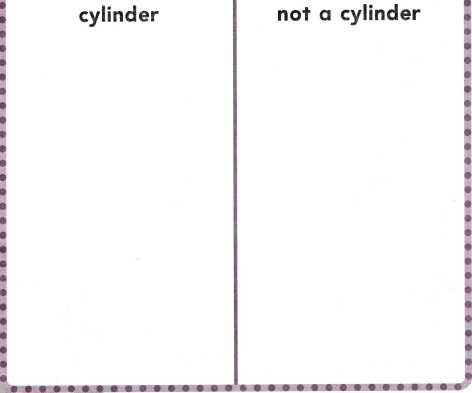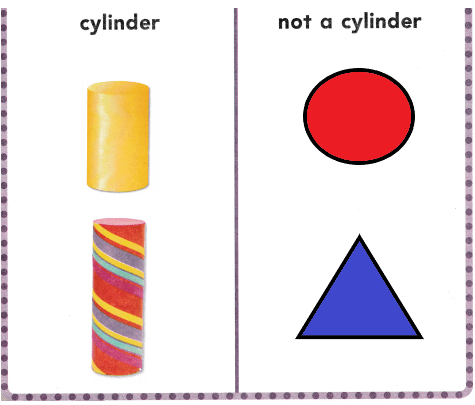Explanation:
I drew some shapes in the above table
Some are cylinders and some are not cylinder.

Directions
Use three-dimensional shapes and identify the cylinder. Sort the shapes. Describe the cylinder. Match a picture of each shape to the shapes on the sorting mat. Glue the shape pictures to show how you sorted

Share and Show

Question 1.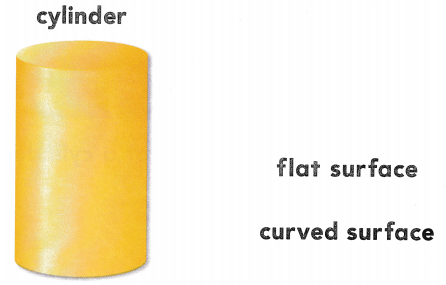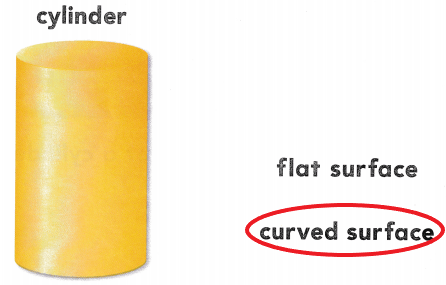Explanation:
I drew circle around the words that describe a cylinder.

Question 2.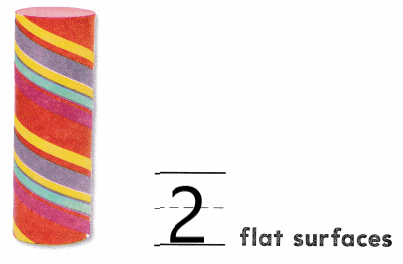Explanation:
A cylinder has 2 flat surfaces
So, i wrote the number 2.

Directions
1. Look at the cylinder. Circle the words that describe a cylinder. 2. Use a cylinder to count how many flat surfaces Write the number.

Question 3.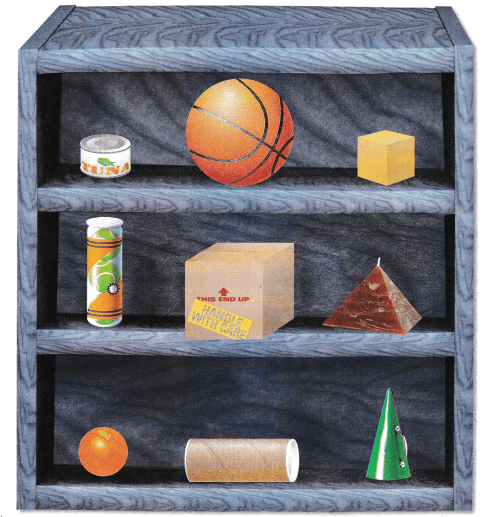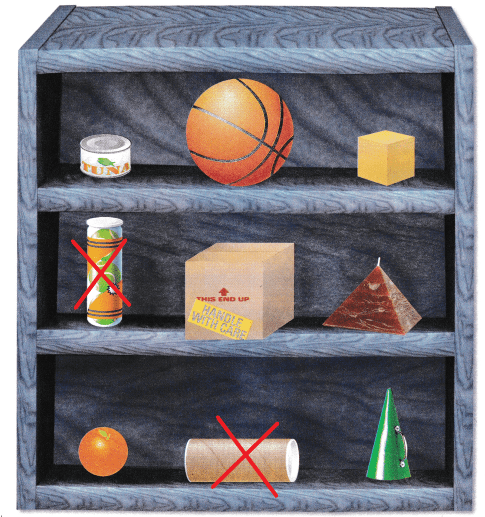Explanation:
I found the cylinder shaped objects in all the objects above
I marked them X.

Directions
3. Identify the objects that are shaped like a cylinder. Mark an X on those objects.

Home Activity

• Have your child identify and describe a household object that is shaped like a cylinder.

Problem Solving

Question 4.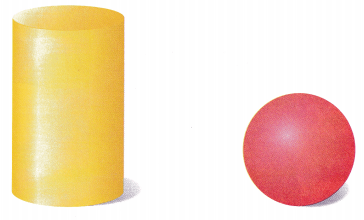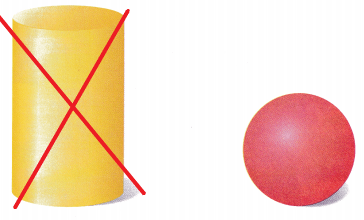Explanation:
I marked X on the shape that has two flat surfaces that are circles.
The shape is a cylinder.

Question 5.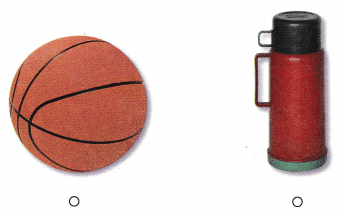Explanataion:
I checked the object that has a shape like a cylinder.

Directions
4. I have two flat surfaces that are circles. Which shape am I? Mark on X on that shape. 5. Choose the correct answer. Which object is shaped like a cylinder?

### Texas Go Math Kindergarten Lesson 18.1 Homework and Practice

Question 1.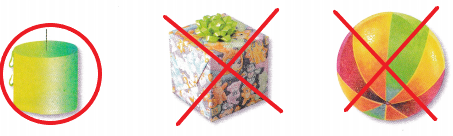Explanation:
I drew a circle around the object that is shaped like a cylinder and marked an X on the objects that are not shaped like a cylinder.

Question 2.Explanation:
I drew a circle around the object that is shaped like a cylinder and marked an X on the objects that are not shaped like a cylinder.

Question 3.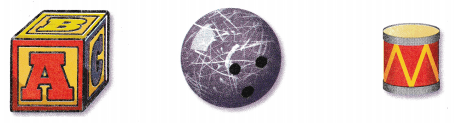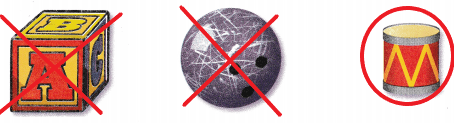Explanation:
I drew a circle around the object that is shaped like a cylinder and marked an X on the objects that are not shaped like a cylinder.

Directions
1-3. Sort the shapes. Circle the object that is shaped like a cylinder. Mark an X on the objects that are not shaped like a cylinder.

Lesson Check

Question 4.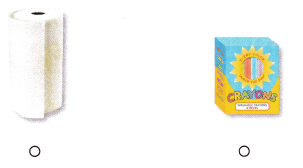Explanation:
I checked the object that looks like a cylinder.

Question 5.Explanation:
I checked the object that has both curved and flat surfaces.

Question 6.